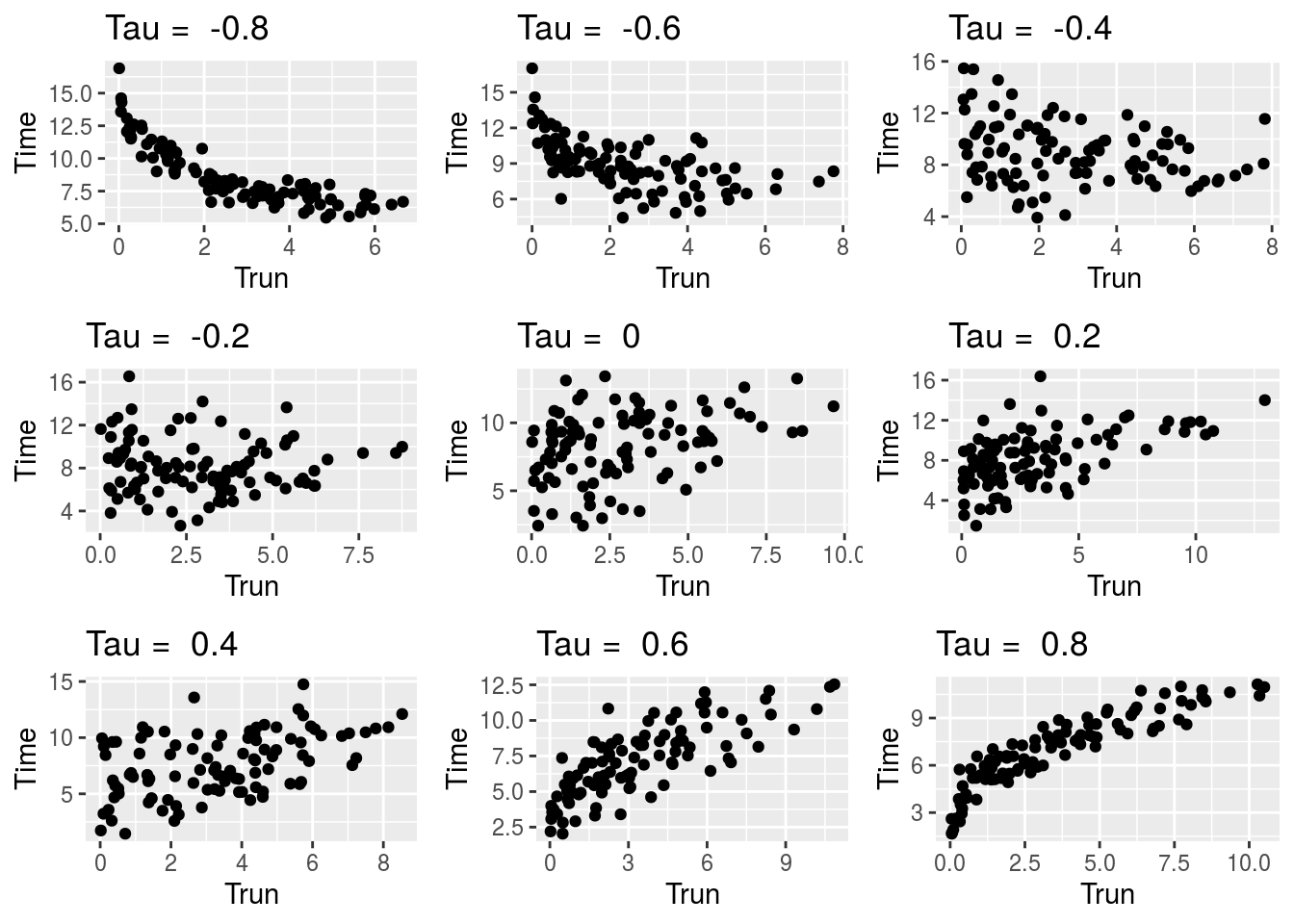## Objective

In this vignette, we demonstrate the usage of the `permDep` function.

## Generating truncated data

We define the following functions to generate survival data with dependent left-truncation.

In the first setting, we generate the survival time from a Weibull distribution with shape parameter 3 and scale parameter 8.5 and generate the truncation time from an exponential with mean 5. The dependence between the survival time and the truncation time is controlled by a normal copula from the `copula` package with a pre-specified pre-truncation unconditional Kendall’s tau (`tau`).

``````> library(copula)
> simDat1 <- function(n, tau) {
+     k <- 1
+     tt <- xx <- rep(-1, n)
+     kt <- iTau(normalCopula(), tau)
+     cp <- normalCopula(kt, dim = 2)
+     dist <- mvdc(cp, c("exp", "weibull"), list(list(rate = 0.2), list(shape = 3, scale = 8.5)))
+     while(k <= n){
+         dat <- rMvdc(1, dist)
+         tt[k] <- dat
+         xx[k] <- dat
+         if(tt[k] <= xx[k]) k = k + 1
+     }
+     data.frame(Trun = tt, Time = xx)
+ }``````

The `simDat1` function generates monotone dependence model and is used in Chiou et al. (2018) in the absence of censoring. The following scatterplots confirms the monotonic dependence.

``````> library(ggplot2)
> library(gridExtra)
> set.seed(123)
> tmp <- lapply(-4:4/5, function(x)
+   qplot(Trun, Time, data = simDat1(100, x), main = paste("Tau = ", x)))
> do.call("grid.arrange", c(tmp, ncol = 3))``````In the second setting, we consider a scenario with non-monotonic dependence between the survival time and the truncation time. We used a normal copula to specify the joint distribution of \((X, |T- 0.5|)\), where \(X\) is the survival time and \(T\) is the truncation time. We generate \(X\) from a Weibull distribution with shape parameter 1 and scale parameter 4, and generate \(T\) from a uniform distribution between 0 and 1. This implies that the pre-truncation unconditional Kendall’s taus have different signs for \(T < 0.5\) and \(T\ge0.5\).

``````> simDat2 <- function(n, tau) {
+     k <- 1
+     tt <- xx <- rep(-1, n)
+     kt <- iTau(normalCopula(), tau)
+     cp <- normalCopula(kt, dim = 2)
+     dist <- mvdc(cp, c("unif", "weibull"),
+                  list(list(min = 0, max = 1), list(shape = 1, scale = 4)))
+     while(k <= n){
+         tmp <- rMvdc(1, dist)
+         if (k <= n/2) tt[k] <- tmp
+         if (k > n/2) tt[k] <- 2 - tmp
+         xx[k] <- tmp
+         if(tt[k] <= xx[k]) k = k + 1
+     }
+     dat <- data.frame(Trun = tt, Time = xx)
+     dat
+ }``````
``````> set.seed(123)
> tmp <- lapply(-4:4/5, function(x)
+   qplot(Trun, Time, data = simDat2(100, x), main = paste("Tau = ", x)))
> do.call("grid.arrange", c(tmp, ncol = 3))``````